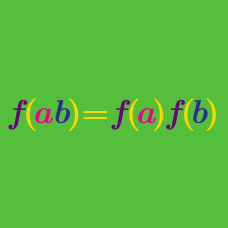Number Theory

# Arithmetic Functions: Level 5 Challenges

If $f: \mathbb{N}\mapsto \mathbb{N}$ is a bijective function that satisfies

$f(xy ) = f(x) f(y)$

and $f(2015) = 42$, what is the minimum value of $f(2000)$?

Find $\large \sum_{d|2016} \mu(d)$ where $\mu$ denotes the Möbius function and the $d$ are the positive divisors of $2016.$

$\sum_{d|n}\mu\left(\frac{n}{d}\right)f(d)=n$

If $f(d)$ is an arithmetic function such that the equation above holds for all positive integers $n$, find $f(2015)$.

Notation: $\mu$ denotes the Möbius function.

$\sum_{\mu(n)=1} \frac{1}{n^2} = \frac{A}{B\pi^C}$

Let $\mu(n)$ denote the möbius function, the sum is taken over all positive integers $n$ such that $\mu(n)=1$, with coprime positive integers $A$ and $B.$ Find $A+B+C$.

Compute

$\large \sum_{d|2015!}\mu(d)\phi(d).$

Notations:

• $\mu(d)$ denotes the Möbius function.
• $\phi(d)$ denotes Euler's totient function.
• $d$ are the positive factors of $2015!.$
×